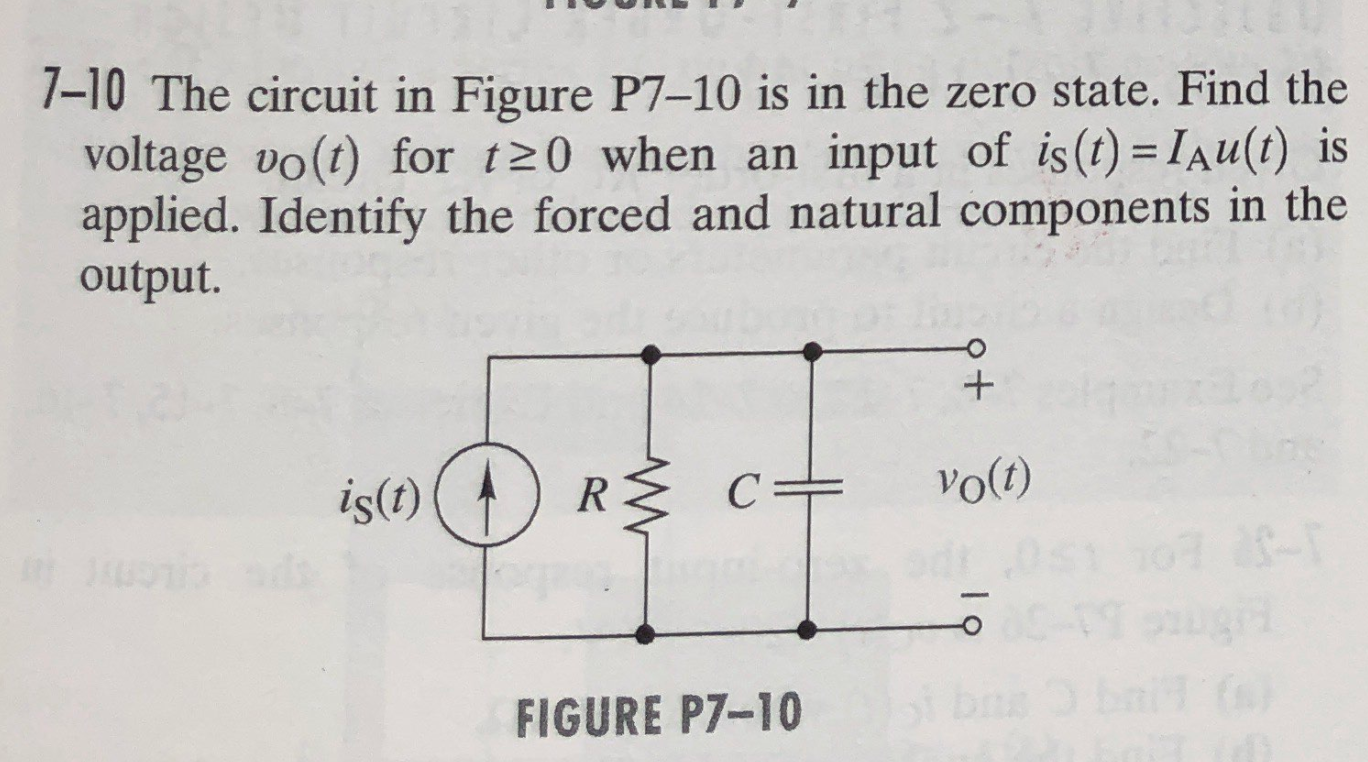# Question llll The circuit in Figure P7-10 is in the zero state. Find the voltage v0(t) for t>0 when an input of is(t) = Iau(t) is applied. Identify the forced and natural components in the output. 7-10 The circuit in Figure P7-10 is in the zero state. Find the voltage vo(t) for 120 when an input of is(t) = lau(t) is applied. Identify the forced and natural components in the output. + is(t) (1RŽ c+ Vo(t) FIGURE P7-10EXLI1Y The Asker · Electrical Engineering

llllThe circuit in Figure P7-10 is in the zero state. Find the voltage v0(t) for t>0 when an input of is(t) = Iau(t) is applied. Identify the forced and natural components in the output.

Transcribed Image Text: 7-10 The circuit in Figure P7-10 is in the zero state. Find the voltage vo(t) for 120 when an input of is(t) = lau(t) is applied. Identify the forced and natural components in the output. + is(t) (1RŽ c+ Vo(t) FIGURE P7-10
More
Transcribed Image Text: 7-10 The circuit in Figure P7-10 is in the zero state. Find the voltage vo(t) for 120 when an input of is(t) = lau(t) is applied. Identify the forced and natural components in the output. + is(t) (1RŽ c+ Vo(t) FIGURE P7-10